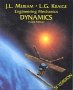# Physics - Kinematics - Angular Acceleration (α)

### Constant Angular Acceleration

When we looked at constant linear acceleration we were able to derive a set of equations to relate the positions, velocities and accelerations:

• v = v0 + a*t
• p = p0 + v0 t + ½ a t2
• v2 = v02 + 2 * a * p

It would be very tempting to just replace the linear values in these equations with their angular counterparts and use those for the equations of constant angular acceleration, unfortunately this would be wrong.

Unlike the linear case, angular orientation behaves differently from the angular velocity and the angular acceleration. Angular accelerations and velocities are combined by vector addition but angular orientations are combined by quaternion or matrix multiplication. In the quaternion case the two different types are related as follows:

d q(t) /dt = ½ * W(t) q(t)

Where:

• q(t) = normalised quaternion representing orientation as a function of time
• W(t) = angular velocity vector represented as quaternion with zero scalar part, i.e
• W (t ) = (0, W x (t ), W y (t ), W z (t ))
• t = time

The above case replaces

dx/dt = v

for the linear case, so we can see that the rotational equations are going to be a more complicated.

At least angular acceleration and angular velocity are both vectors (bivectors) so we can start off, in the same way that we did for the linear case, by integrating the constant acceleration:

α = dω/dt = constant

integrating both sides:

∫α dt = ωf - ωi = α * t

 where: other definitions symbol description type units α instantaneous angular acceleration about centre. bivector s-2 ωi initial instantaneous angular velocity about centre. bivector s-1 ωf final instantaneous angular velocity about centre. bivector s-1 t time scalar s

But now we want to relate this to the orientation which we do by integrating again, but this time we have to be careful, using the earlier equation:

d q(t) /dt = ½ * W(t) q(t)

substituting W(t) = α * t gives:

d q(t) /dt = ½ * α * t * q(t)

I am not sure how to continue this to get the angular equivalent of p = p0 + v0 t + ½ a t2 because in the above case q(t) appears twice.

### Dynamics Issues

In the linear case, constant acceleration happens in a constant gravity field where there is no friction, due to F=m*a.

So when would constant angular acceleration be likely to happen? We can imagine cases where the torque may be made constant, for example:

• An aeroplane with control surfaces.
• A spacecraft with maneuvering jets.

However unlike the linear case, the relationship between torque and angular acceleration is given by:

T = [I]α

where [I] is the inertia tensor (as explained on this page), this inertia tensor may be a function of orientation and therefore of time which would also make α a function of time.

Where I can, I have put links to Amazon for books that are relevant to the subject, click on the appropriate country flag to get more details of the book or to buy it from them.Engineering Mechanics Vol 2: Dynamics - Gives theory for rigid dynamics, aims to allow prediction of effects of force and motion. Includes rotating frame of reference. Lots of colour diagrams, I guess its college / University level.

Commercial Software Shop

Where I can, I have put links to Amazon for commercial software, not directly related to the software project, but related to the subject being discussed, click on the appropriate country flag to get more details of the software or to buy it from them.Dark Basic Professional Edition - It is better to get this professional editionThis is a version of basic designed for building games, for example to rotate a cube you might do the following: make object cube 1,100 for x=1 to 360 rotate object 1,x,x,0 next x

This site may have errors. Don't use for critical systems.